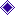Michael Coins LtdHomeEx-ShopAbout MichaelTermsGradingCode & RefGB Coin listExtras !British BanknotesOur Books, etcWorld CoinsWorld BanknotesAncientsOther ListsRecord & Video

COIN CLUBReading Coin Club

Ref Type Series Date Denomination

Prefix

Price (£)

J.Q. Hollom - 1st issue (l~~l)
B288 B~~N Start 1963 One Pound - B98N EF 50.00
B288 l~~l Mid 1963 One Pound - H~~N
Run of 4 - Sold as pair or + (each) EF+ 7.50
B288 l~~l Mid 1963 One Pound - K~~U
Run of 6 - Sold as pair or + (each) EF+ 7.50
B288 l~~l Mid 1963 One Pound - H~~W; J~~X
- L~~U
Consecutive pair.   Sold as pair EF+ 15.00
B288 l~~l Mid 1963 One Pound - A~~Y; B~~U: B~~X
- C~~S; C~~U; C~~X
- E~~S; E~~T; E~~U
- H~~X; L~~R; L~~U EF+ 7.50
B288 l~~l Mid 1963 One Pound - B~~X
Run of 4 - Sold as pair or + (each) EF-EF+ 6.00
B288 l~~l Mid 1963 One Pound - A~~S; A~~X
- B~~X; C~~T; C~~X
- D~~X; E~~N; E~~R
- E~~S: H~~N: H~~R
- H~~T; H~~X; J~~S
- K~~T; L~~T; L~~U EF-EF+ 6.00
B288 l~~l Mid 1963 One Pound  - Too many to list !
If you require a certain prefix let us
know and we will look if we have it EF 4.50
B288 l~~l Mid 1963 One Pound EF? 3.50
B288 B~~Y End 1963 One Pound - B06Y; B08Y EF 50.00
 J. Q. Hollom   Replacement  (M~~)  1st issue
B289 M~~ Mid 1963 One Pound - M71 EF-EF+ 45.00
B289 M~~ Mid 1963 One Pound - M74: N79; M85 EF 35.00
B289 M~~ Mid 1963 One Pound - M92 VF+ 25.00
 J. Q. Hollom   Replacement  (~~M)  2nd issue
B290 M~~ Mid 1963 One Pound - 03M EF+ 65.00
B290 M~~ Mid 1963 One Pound - ~~M EF+ 40.00
B290 M~~ Mid 1963 One Pound - ~~M EF-EF+ 30.00
B290 M~~ Mid 1963 One Pound - 25M
100 in ink - front left EF-EF+ 15.00
B290 M~~ Mid 1963 One Pound - ~~M EF/VF 10.00
B290 M~~ Mid 1963 One Pound - ~~M EF/VF? 7.50
B290 M~~ Mid 1963 One Pound - 96M EF 50.00
J.Q. Hollom - printed on a Goebel machine (G on rev.)
B292 A~~N Start 1963 One Pound - A77N
Run of 6 - Sold as pair or + (each) EF+ 22.50
B292 A~~N Start 1963 One Pound - A~~N EF+ 22.50
B292 A~~N Start 1963 One Pound - A~~N EF-EF+ 17.50
B292 A~~N Start 1963 One Pound - A~~N EF 12.50
B292 A~~N Start 1963 One Pound - A~~N EF/VF+ 5.00
B292 C~~W Mid 1963 One Pound - C~~W EF+ 17.50
B292 C~~W Mid 1963 One Pound - C~~W EF-EF+ 12.50
B292 C~~W Mid 1963 One Pound - C~~W EF 7.50
B292 D~~T Mid 1963 One Pound - D~~T EF+ 17.50
B292 D~~T Mid 1963 One Pound - D~~T EF-EF+ 12.50
B292 D~~T Mid 1963 One Pound - D~~T EF 7.50
B292 L~~X End 1963 One Pound - L22X
Consecutive pair.   Sold as pair EF+ 45.00
B292 L~~X End 1963 One Pound - L22X EF+ 22.50
B292 L31X End 1963 One Pound - L31X    EF-EF+ 50.00
J.Q. Hollom - Goebel Replacement notes (G on rev.)
B293 M~~N Only 1963 One Pound M~~N EF+ 80.00
B293 M~~N Only 1963 One Pound M~~N EF-EF+ 65.00
 British Banknotes Hollom 10/- Hollom £5 & £10